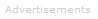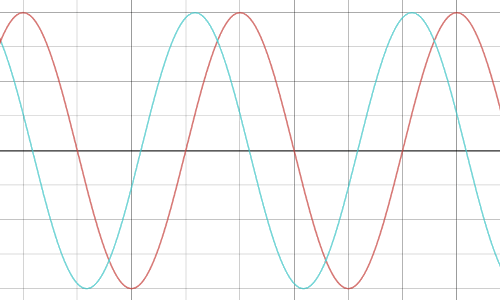# Definition of Impedance

What is Impedance?

Impedance is a frequency dependent measure of the opposition to current flow in an electric circuit. Since impedance is frequency dependent it is only observed in AC circuits.Like electrical resistance, impedance is measured in ohms, symbol Ω. In fact, electrical resistance can be viewed as a special case of impedance.

A perfect resistor's behavior does not depend on the electricity's frequency. When an AC voltage is applied to an ideal resistor, the current flowing is always in phase with the voltage - in other words, regardless of the frequency, voltage and current peak and trough at the same time: the ratio of voltage:current is constant.

When there is impedance rather than resistance, the current flowing is out of phase with the voltage: the ratio of voltage:current depends on frequency.When there is impedance, the peaks and troughs of the voltage (red) and current (cyan) are out of phase. In this case, the current lags behind the voltage.

Mathematically, phase differences are most readily dealt with using complex numbers. Impedance, Z, can be calculated from the equation:

Z = R + jX

where R is resistance, j is √-1, and X is reactance.

The reactance - the frequency dependent part of impedance - of different electrical devices varies. The reactance of an inductor, ZL, is:

ZL = jωL

where j is √-1, ω is frequency, and L is inductance.

The reactance of a capacitor, ZC, is:

ZC = (jωC)-1

where j is √-1, ω is frequency, and C is capacitance.

Most electrical systems are not purely resistive. In the real world there is usually impedance whether deliberately or accidentally.

A pure resistance opposes current by dissipating energy as heat. An inductor opposes current by storing energy in a magnetic field. When current falls, the inductor releases its stored energy. A capacitor opposes current by storing energy in an electric field. When voltage falls, the capacitor releases its stored energy.Search the Dictionary for More Terms